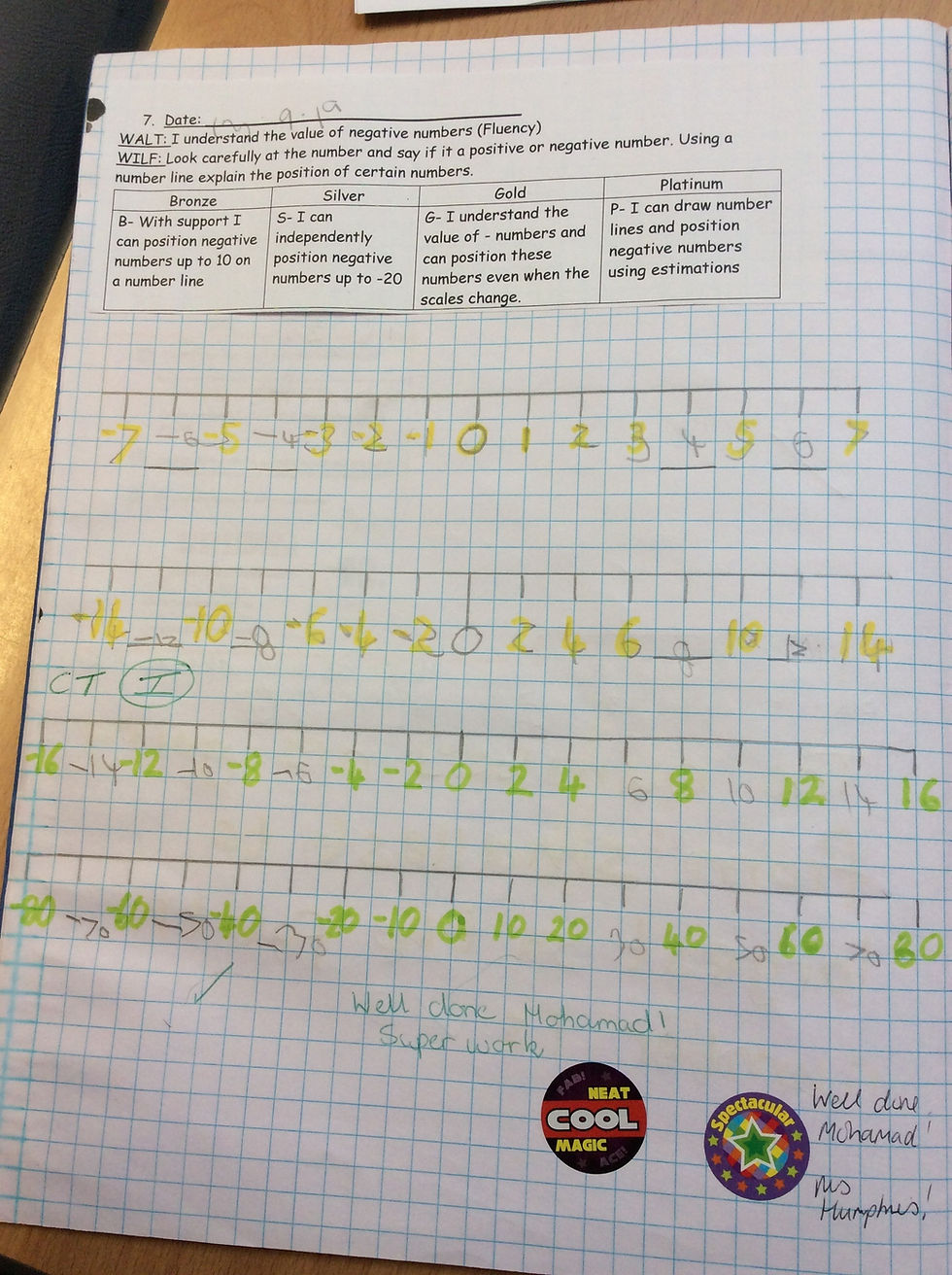top of page# Maths

This term Year 4 have been looking at the following topics in Maths.

Place Value

This term, we have focused on the value of numbers and partitioning numbers to show the value of each number.

Place Value

We have also looked at Roman numerals - these are important because they are still used in many areas of life still to this day.

Test you child on their knowledge at home by following this link

Negative Numbers

We use a number line in Year 4 to help us to work with negative numbers. Here is an example of some of the work we produced.Knowing how to add and subtract using standard written method (SWM) is very important in KS2.

Perimeter

To start our amazing maths learning in Autumn 2, we had an awesome practical maths lesson outside! We created shapes using cones, and measured the perimeter using trundle wheels. This really helped us to understand the concept of perimeter. If you would like to create your own shapes and work out the perimeter, just follow the link.

Working out perimeter

Multiplying and Dividing by 10 and 100

Over the past two weeks, we have been learning to multiply and divide by 10 and 100.

Check out the link below to have a go!

Moving Digits

This week in maths we have been learning our 6, 7 and 9 times table.

Click the links below to to sing and dance along with the Supermovers!

6 x table with Fred the Red

7 x table with Moonbeam

9 x table with Rapping Unicorn

See All
bottom of page